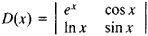# Functional Determinant

Also found in: Wikipedia.

## Functional Determinant

a determinant whose elements are functions of one or more variables.

The most important examples of functional determinants are the Wronskian, which plays an important role in the theory of linear differential equations of higher order, the Hessian, which is used in the theory of algebraic curves, and the Jacobian, which is used in transforming multiple integrals, in establishing the independence of a system of functions, and in other problems of the theory of functions of several variables.

The derivative of a functional determinant D(x) = |aik(x)| of order n is the sum of the h functional determinants whose matrices are obtained from the matrix ‖aik(x)‖ by differentiation of the elements of the first, second, . . ., nth column. For example, ifthenThe term “functional determinant” is sometimes used to refer to the Jacobian.

Site: Follow: Share:
Open / Close